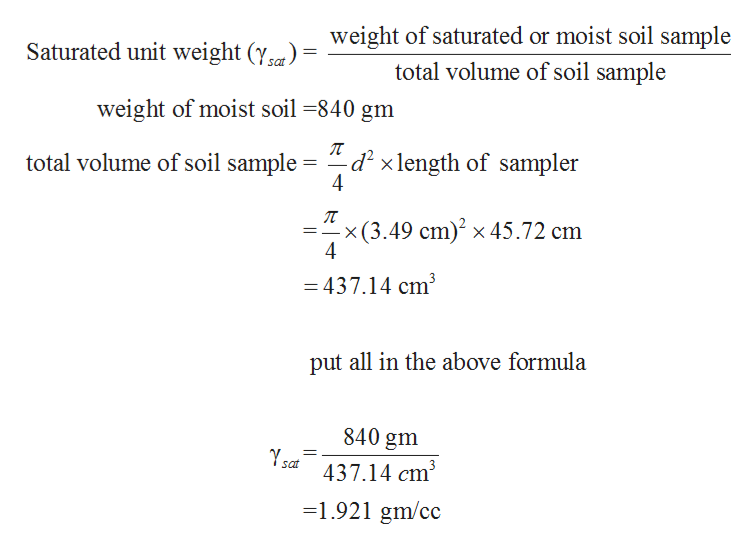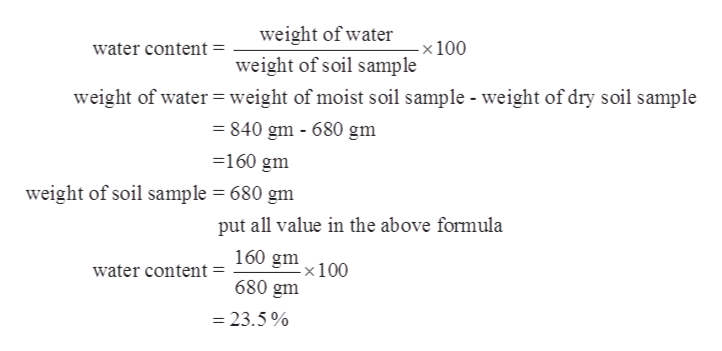# during a subsurface exploration, an undiscovered soil sample was collected from the field using a split spoon sampler for lab evaluation. the sample has a diameter of 34.9mm length of 457.2mm and a moist mass of 840g. if the oven dried mass was 680g and Gs= 2.74 calculate the ff.a.)moist unit weightb.)moisture contentc.)dry unit weightd.)void ratioe.)degree of saturation

Question
26 views

during a subsurface exploration, an undiscovered soil sample was collected from the field using a split spoon sampler for lab evaluation. the sample has a diameter of 34.9mm length of 457.2mm and a moist mass of 840g. if the oven dried mass was 680g and Gs= 2.74 calculate the ff.

a.)moist unit weight

b.)moisture content

c.)dry unit weight

d.)void ratio

e.)degree of saturation

check_circle

Step 1

Given Data:

Undisturbed Sample of Soil is given

Volume of Soil Sample = Volume of split spoon sampler

Diameter of Sampler = 34.9 mm

Length of sampler = 457.2 mm

Moist mass of Soil = 840 gm

Dry mass of Soil = 680 gm

Specific Gravity of Soil (Gs) = 2.74

Step 2

Calculating moist unit weight.help_outlineImage Transcriptioncloseweight of saturated or moist soil sample Saturated unit weight (Ysa= total volume of soil sample weight of moist soil -840 gm -d2xlength of sampler 4 total volume of soil sample * x(3.49 cm)2 x 45.72 cm =437.14 cm3 put all in the above formula 840 gm sat 437.14 cm3 1.921 gm/cc fullscreen
Step 3

Calculating moisture conte...help_outlineImage Transcriptioncloseweight of water x 100 weight of soil sample water content = weight of water weight of moist soil sample - weight of dry soil sample 840 gm - 680 gm 160 gm weight of soil sample = 680 gm put all value in the above formula 160 gm -x 100 water content = 680 gm - 23.5% fullscreen

### Want to see the full answer?

See Solution

#### Want to see this answer and more?

Solutions are written by subject experts who are available 24/7. Questions are typically answered within 1 hour.*

See Solution
*Response times may vary by subject and question.
Tagged in
EngineeringCivil Engineering

### Geotechnical Engineering